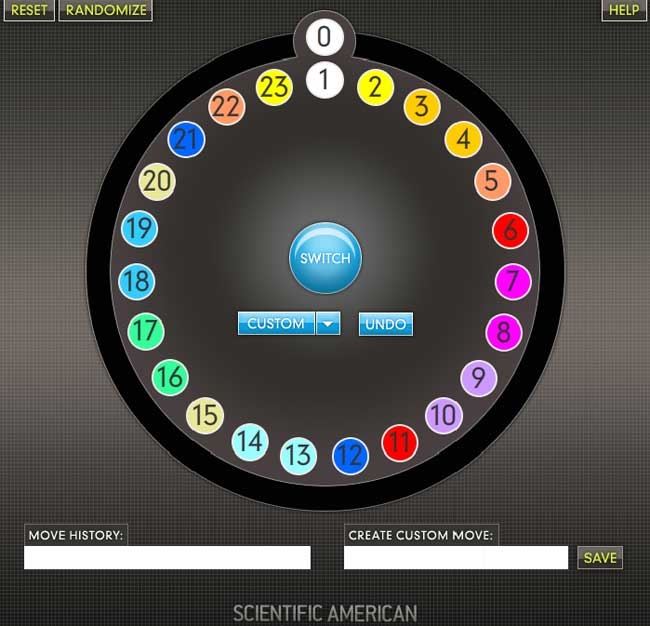# Diameter of the M24 Conway puzzle is 45This is a well know puzzle in which there are two moves, one which rotates the central "clock" by one position clockwise or counter-clockwise.
The other switches or swaps each pair of numbers with matching colours.

I decided to plug these values into GAP to investigate God's number for the underlying group for this puzzle
as it still seemed be unknown or undocumented anywhere at least. Feeding these numbers into GAP we get:

S := (1,24)(2,23)(3,4)(5,22)(6,11)(7,8)(9,10)(12,21)(13,14)(15,20)(16,17)(18,19);
R := (1,2,3,4,5,6,7,8,9,10,11,12,13,14,15,16,17,18,19,20,21,22,23);

Note that we have the number 24 in place of 0 to be GAP compatible.

Table: Distribution of word count in the Cayley graph for M24 Conway puzzle
```Len  Count
0    1    (the identity)
1    3    (R, R^-1 and S)
2    6    {R^2, R^-2, R*S, S*R, R^-1*S, S*R^-1}
3    10
4    16
5    26
6    42
7    68
8    110
9     178
10    288
11    466
12    752
13    1214
14    1958
15    3132
16    4980
17    7957
18    12738
19    20375
20    32563
21    52010
22    83000
23    132360
24    210896
25    335265
26    531855
27    842722
28    1332401
29    2098880
30    3291324
31    5121482
32    7872753
33    11885315
34    17455355
35    24565715
36    32401139
37    38652273
38    39596760
39    32213183
40    18556076
41    6407846
42    1041497
43    55472
44    577
45    1
```

So there is exactly one node requiring a manouevre of length 45 in the Cayley graph for the group generated by R and S
assuming my results are correct. It would be nice to have independent verification of of the results in the table here
(even up to a certain length).

Expressed in term of the generators the single node w of length 45 is:

w := R^3*S*R^-4*S*R^2*S*R^-1*S*R*S*R^-2*S*R*(R^2*S*R^-4*S)^2*R^2*S*R^-3*S*R*S;

It is the permutation (2,19)(6,20)(7,16)(8,17)(11,15)(12,13)(14,21)(18,23). This differs from the
original switch (S) in that 4 pairs of transpositions are omitted, and 8 remaining pairs of transpositions
(colours) get swapped around (see diagram above to visualise).

For the enthusiasts only! We may take in turn the group generated by w and R and we have a free of charge puzzle for the point stabiliser of M24
(namely M23) with the point 24 (or zero as originally put forward) omitted using the same diagram. This in turn has so-called
God's number 32 with 11 nodes at the diameter - the odd one out of which is the involution

R^3*w*(R*w*R)^2*R^4*(w*R^-1)^2*R^-1*w*R*(w*R^-1)^2*(w*R)^3*w

Here is a snippet of the GAP code I used to preform the M24 Conway puzzle Cayley graph calculations:

M24:=Group(S,R);
w:=(2,19)(6,20)(7,16)(8,17)(11,15)(12,13)(14,21)(18,23);
f:=Factorization(M24,w); /* Needed as this function can otherwise return suboptimal results */
arr:=[];
for i in [1..45] do; arr[i]:=0;od;
n:=Iterator(M24);
for i in [1..244823040] do;
a:=NextIterator(n);
f:=Factorization(M24,a);
l:=Length(f);
if l>0 then arr[l]:=arr[l]+1;fi;
if Length(f) > 43 then Print(f, "-", Length(f),"-",i,"\n");fi;od;

## Comment viewing options

### Confirmed

I have confirmed your numbers with twsearch (and added m24conway.tws to the samples).

I've also run it permitting any distance rotation on R, and got these counts:

0 1
1 23
2 44
3 504
4 878
5 9551
6 16632
7 179880
8 312092
9 3333993
10 5686316
11 53267905
12 65375126
13 115590954
14 1048727
15 414

Further, your antipode can be solved with this simpler and nicely symmetrical sequence, with only six swaps:

S R9' S R11 S R11' S R11 S R11' S R9

-tom

### Errata

The diameter for the Mathieu group M23 I have outlined is 27 (and not 32 as I posted in error)
with 11 nodes at the diameter, one of which is the following involution:

R^2*(w*R^-1)^2*w*R*(R^3*w)^3*R^-2*w*R*w*R^-1*w

For info this is the permutation:

(2,12)(6,14)(7,23)(8,15)(11,17)(13,19)(16,18)(20,21)